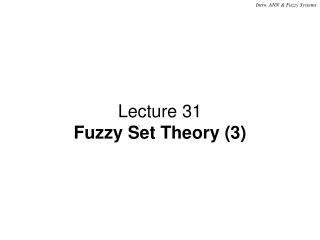DownloadDownload PresentationLecture 31 Fuzzy Set Theory (3)

# Lecture 31 Fuzzy Set Theory (3)

Download Presentation## Lecture 31 Fuzzy Set Theory (3)

- - - - - - - - - - - - - - - - - - - - - - - - - - - E N D - - - - - - - - - - - - - - - - - - - - - - - - - - -
##### Presentation Transcript

1. Lecture 31 Fuzzy Set Theory (3)

2. Outline • Fuzzy Relation Composition and an Example • Fuzzy Reasoning (C) 2001 by Yu Hen Hu

3. Fuzzy Relation Composition • Let R be a fuzzy relation in X  Y, and S be a fuzzy relation in Y  Z. • The Max-Min composition of R and S, RoS, is a fuzzy relation in X  Z such that RoS  µRoS(x,z) =  {µR(x,y)  µS(y,z) } = Max. {Min. {µR(x,y), µS(y,z)}}/(x,z) • The Max-Product Composition of R and S, RoS, is a fuzzy relation in X  Z such that RoS  µRoS(x,z) =  {µR(x,y)  µS(y,z) } = Max. {µR(x,y) µS(y,z)}/(x,z) (C) 2001 by Yu Hen Hu

4. Fuzzy Composition Example • Let the two relations R and S be, respectively: • The goal is to compute RoS using both Max-min and Max-product composition rules. (C) 2001 by Yu Hen Hu

5. MAX-MIN Composition RoS = max{min(0.4,0.5), min(0.6, 0.1), min(0, 0)} = max{ 0.4, 0.1, 0} = 0.4 max{min(0.4,0.8), min(0.6, 1), min(0, 0.6)} = max{ 0.4, 0.6, 0} = 0.6 max{min(0.9,0.5), min(1, 0.1), min(0.1, 0)} = max{ 0.5, 0.1, 0} = 0.5 max{min(0.9,0.8), min(1, 1), min(0.1, 0.6)} = max{ 0.8, 1, 0.1} = 1 (C) 2001 by Yu Hen Hu

6. MAX-PRODUCT Composition • max{0.40.5, 0.60.1, 00} = max{0.02,0.06,0} = 0.06 • max{0.40.8, 0.61, 00.6} = max{0.32, 0.6, 0} = 0.6 • max{0.90.5, 10.1, 0.10} = max{0.45, 0.1, 0} = 0.45 • max{0.90.8, 11, 0.10.6} = max{0.72, 1, 0.06} = 1 (C) 2001 by Yu Hen Hu

7. Fuzzy Reasoning • Comparing crisp logic inference and fuzzy logic inference Translation – Age(Mary) = 22 (Age(Dana),Age(Mary)) = Age(Dana)–Age(Mary) = 3 \ Age(Dana) = Age(Mary) + 3 = 22 + 3 = 25 (C) 2001 by Yu Hen Hu

8. Fuzzy Reasoning • Translation – • Age(Mary) = Young (Young is a fuzzy set) • (Age(Dana),Age(Mary)) = Much_older (a relation) • \ Age(Dana) = Young o Much_older • – a composite relation! (C) 2001 by Yu Hen Hu

9. Fuzzy Reasoning (cont'd) • µAge(Dana)(x) =  {µyoung(y)  µmuch_older(x,y) } The universe of discourse (support) is "Age" which may be quantified into several overlapping fuzzy (sub)sets: Young, Mid-age, Old with the following definitions: (C) 2001 by Yu Hen Hu

10. Fuzzy Reasoning (cont'd) • Much_older is a relation which is defined as: µmuch_older(x,y) = (C) 2001 by Yu Hen Hu

11. Reasoning Example For each fixed x, find µAge(Dana)(x) = max(min(µyoung(y),µmuch_older(x,y)): (C) 2001 by Yu Hen Hu